SYLLABUS  Previous: 5.2 Credit derivatives  Up: 5.2 Credit derivatives  Next: 5.2.2 Cap-/floorlets

### 5.2.1 Vanilla swaps

[ SLIDE model - experiments || VIDEO login]

Remember from sect.2.2.3 that a swap is a contract derived from a loan, where the payments from a fixed interest rate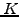are exchanged for the payments from a floating interest rate. In a plain vanilla swap, the floating rate is evaluated at the end of every accrual period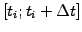when a payment is made in compensation for the difference in rates.

To avoid difficulties with a floating rate that is only known at the end of the accrual period, the calculation proceeds backwards in time and evaluates the price (of what is sometimes called FRA) for each period separately using the same procedure as for bonds. The equilibrium swap rateis chosen so as to make the contract worthless at the onset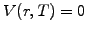and the mismatch between the spot rate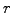and the swap rateis accumulated over the accrual period to calculate the price of a swap having a finite lifetime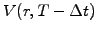. For a unit Notional principal, the incremental change in swap value is the difference of interest rates multiplied by the time interval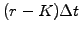. As for any asset with an investment value, the accumulated earnings or losses from the swap can themselves be viewed as bonds with a positive or negative value and can therefore be described using the Vasicek model from the previous section. In fact, a swap can be understood as a bond that starts with zero as initial value and pays a continuously compounded annual coupon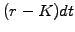. Only one parameter is required in addition to those that have been defined in sect.5.1.2:

• the fixed swap rate (or StrikePrice) is expressed as the relative annual return in the fixed leg, e.g. 0.04 for a predetermined swap rate of 4%.

The VMARKET applet below shows how the value of a swap with a fixed rate of 8% evolves as a function of the spot rate for an increasing time to the maturity.

Immediately after the start of the contract, the swap acquires a negative value if the spot rate is below the swap rate because the holder of the swap has the obligation to pay the fixed rate: this is indeed more than the market is asking for and the swap holder is therefore loosing money to the writer of the swap. On the contrary, the swap acquires a positive value if the spot rate is above the swap rate: the swap holder has the right to pay only a fixed rate and is therefore earning money at the expense of the writer.

 Virtual market experiments: a simple swap Change the swap rate (or StrikePrice) and observe what happens. Explain the existence of a fixed point, for which the swap remains worthless. Why does the swap value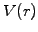bend downwards after some time?

Think of a swap as a coupon paying bond: the downward curvature of the price (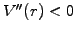) is the results of the exponential growth at the spot rate, which is expected for any risk free investment when the time runs forward. The opposite happens when the time is reversed and the exponential decrease of the swap price with the spot rate results in a downward curvature in the same manner as previously seen for the discount function.

The same models that have been used for bonds forecast the drift in the interest rate, but the volatility should here be modified to reflect the uncertainty of payments in the floating leg (exercise 5.07). The volatility reduces the overall curvature and therefore also reduces the value of the swap: this can be understood financially from the spot rate fluctuations above and below the swap rate, which tend to cancel out in time and reduce the value of the swap.

 Virtual market experiments: forecasting rates and volatility for a swap Study the effect of a typical Volatility=0.1 observed in swap markets. Account for a finite market price of risk MktPriceRsk or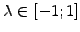and explain the movement of the neutral point where the swap is worthless. Examine the price of a swap during economic cycles setting UserDouble=2. Reload the default parameters and adjust mean reversion parameters to reproduce a drift (5.1.1#eq.5) proportional to the spot rate. Explain why the value of the swap becomes a linear function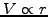.

SYLLABUS  Previous: 5.2 Credit derivatives  Up: 5.2 Credit derivatives  Next: 5.2.2 Cap-/floorlets